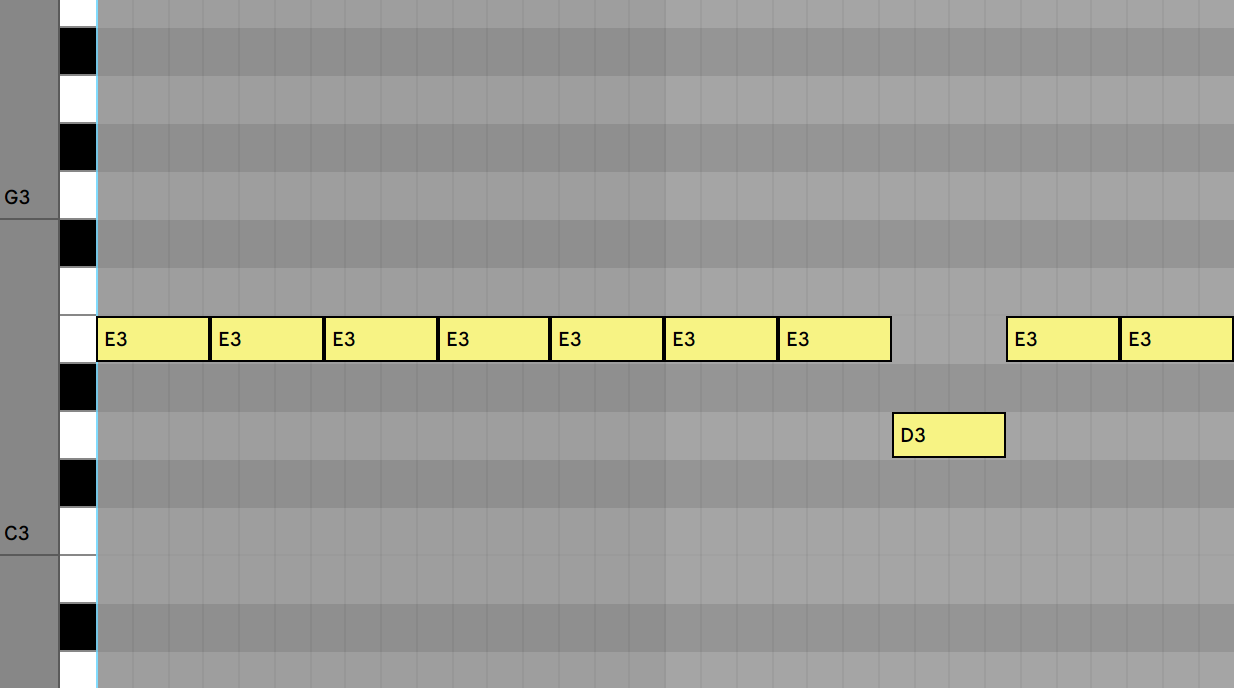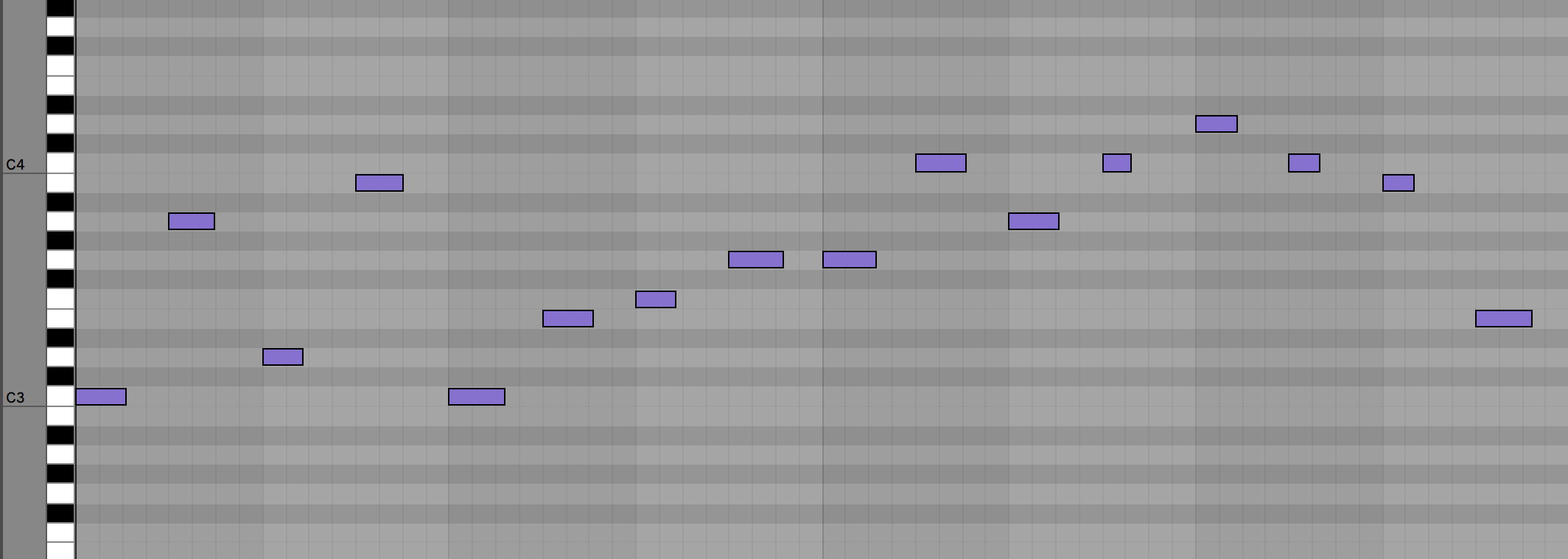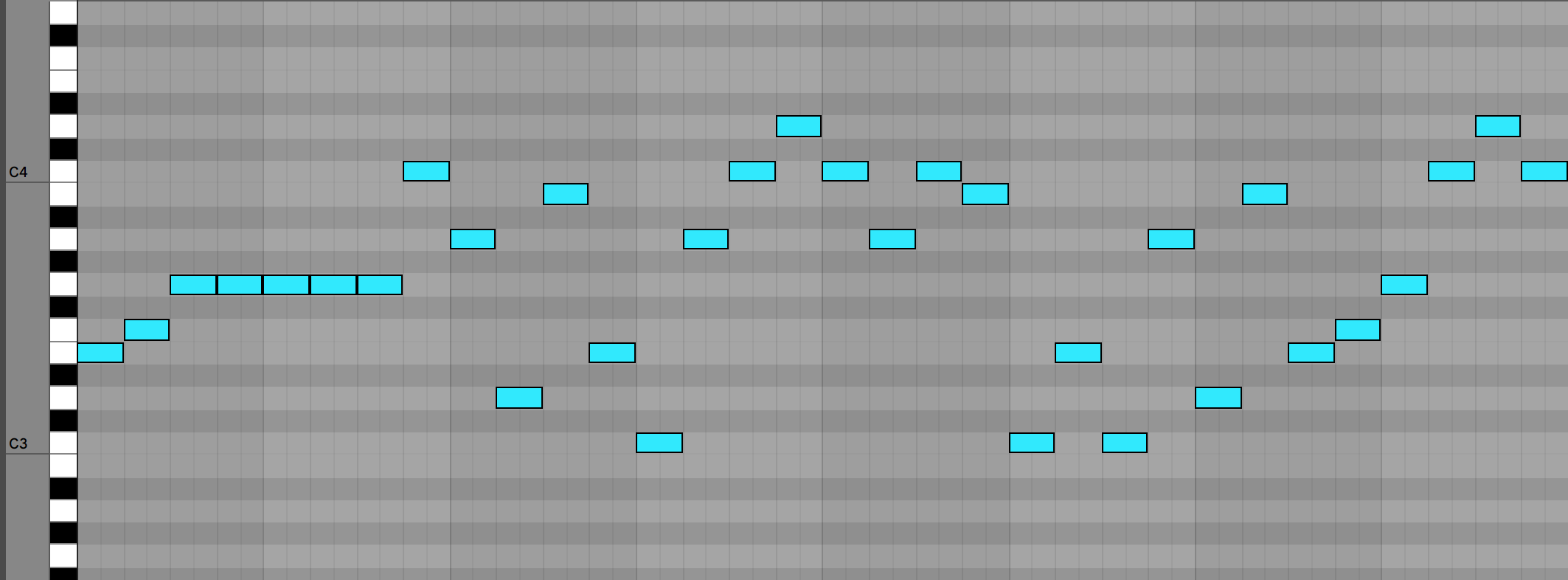Let’s write some code to generate melodies with Markov Chains.

## What’s a Markov Chain?

A Markov Chain is essentially a finite state machine that we can get to generate output based on probability from previous input. It’s probably one of simplest generative systems. It’s especially fun to use for things like generating sentences that sound semi-coherent from gigantic inputs like a Shakespeare play.

Let’s get an array of notes from a MIDI file with `midilib`.

``````require 'midilib'

# Create a new, empty sequence.
seq = MIDI::Sequence.new()

# Read the contents of a MIDI file into the sequence.
File.open('simple melody.mid', 'rb') { | file | seq.read(file) }
``````

Now we have a `seq` object to read events from. Let’s get the events.

``````events = []

events = seq.map do |track|
track.map { |e| e }
end
``````

Now we have an array of event objects we can work with. Here’s a snippet:

``````48: ch 00 on 3c 64
48: ch 00 off 3c 40
``````

`midilib` gives us a lot of information. For each note, it looks there is an event for on and an event for off. Since a note is represented by two events, for simplicity’s sake let’s just worry about the ‘On’ Midi events and make their length a quarter note. We should probably make our own note object that will make it easier to work with for predicting future notes.

``````class Note
attr_accessor :position, :note, :length

def initialize(position, note, length)
@position = position
@note = note
@length = length
end

def to_s
"#{@position}, #{@note}, #{@length}"
end
end
``````

Let’s create a list of these simplified note representations to feed into our Markov Chain.

``````quarter_note_length = seq.note_to_delta('quarter')

notes = []

events.first.each do |event|
if event.kind_of?(MIDI::NoteOn)
note = Note.new(event.time_from_start, event.note, quarter_note_length)
notes << note
end
end
``````

This is an extremely simplistic representation of notes. It doesn’t even have velocity. But it is ordered and thus we can create a Markov Chain from it.

Now let’s create a hash of the frequencies, based on the note being played. The key will be the note and the value will be an array of notes played after that note.

``````frequencies = Hash.new { |h, k| h[k] = [] }

notes.each_cons(2) do |w1, w2|
frequencies[w1.note] << w2
end

# Make the last note loop back to the first
frequencies[notes.last.note] << notes.first
``````

Now, we have a simple hash based on notes that can show us what the next note will likely sound like! Here is a snippet:

``````{60=>
[#<Note @length=96, @note=60, @position=96>,
#<Note @length=96, @note=60, @position=192>,
#<Note @length=96, @note=62, @position=240>],
62=>
[#<Note @length=96, @note=64, @position=336>,
...
``````

So, for example, note id `60` (which is E3) will either play note id `60` again, or note `62`. Since note ID `60` shows up twice, it’s the more likely contender. Then, once `62` is chosen, we have a new array of notes that could be chosen.

So now we have Markov Chain of notes! Now, let’s generate an array from this.

``````generated = [notes.sample]

for i in 0..32 do
next_note = frequencies[generated.last.note].sample
generated << next_note
end
``````

Which prints out:

``````624, 67, 96
720, 69, 96
0,   60, 96
240, 62, 96
336, 64, 96
432, 64, 96
432, 64, 96
528, 64, 96
576, 62, 96
``````

Whoops. Looks like we’re generating notes with the wrong time. We want to increment the time every time we’ve done this.

``````generated = [notes.sample]

for i in 0..32 do
next_note = frequencies[generated.last.note].sample
next_note.position = i * quarter_note_length
generated << next_note
end
``````

So, now, we need to convert this back into a playable file.

``````# Generate the midi
seq = Sequence.new()

track = Track.new(seq)
seq.tracks << track
track.events << Tempo.new(Tempo.bpm_to_mpq(120))
track.events << MetaEvent.new(META_SEQ_NAME, 'Markov Type Beat')

# Create a track to hold the notes. Add it to the sequence.
track = Track.new(seq)
seq.tracks << track

# Add a volume controller event (optional).
track.events << Controller.new(0, CC_VOLUME, 127)

track.events << ProgramChange.new(0, 1, 0)
quarter_note_length = seq.note_to_delta('quarter')

generated.each do |generated_note|
track.events << NoteOn.new(0, generated_note.note, 127, 0)
track.events << NoteOff.new(0, generated_note.note, 127, quarter_note_length)
end

File.open('from_scratch.mid', 'wb') { |file| seq.write(file) }
``````

Thanks midilib documentation.

Here’s an example of a generated melody based on the very simplistic inputted MIDI file.Lots of E3s because of the input!

Let’s give it a more varied input and generate.

Input (Four Bars):Output (Eight Bars):As you can see, there are more notes here because we’re outputting quarter notes only with no rests. Looks like the Markov Chain got caught on the G note a few more times than probably is sonically pleasing.

This is code is much more applicable to melodies than it is to a song structure or a chord progression. It would be fun to expand on this further with a few things:

• Give generator the concept of rests
• Give notes the concept of velocity
• Chords
• Octave awareness (for chord inversions)
• More meaningful user input or browser interactivity

I like this because it allows me to sort of jam with the computer. I can play a melody, and then hear various variations close to its style outputted by the code. From there, I can tweak it and feed it back into the program to get something more interesting.

Have you done anything interesting with generative music? I’d love to hear from you! Email me at mattbettinson@gmail.com. Get the source code here.